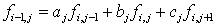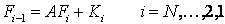## Option Pricing Using The Explicit Finite Difference Method

This tutorial discusses the specifics of the explicit finite difference method as it is applied to option pricing. Example code implementing the explicit method in MATLAB and used to price a simple option is given in the Explicit Method - A MATLAB Implementation tutorial.

The Finite Difference Methods tutorial covers general mathematical concepts behind finite diffence methods and should be read before this tutorial. Alternative finite difference methods, namely the implicit method and the Crank-Nicolson method, are covered in companion tutorials.

### Discretizing the Black-Scholes-Merton PDE

For the explicit method the Black-Scholes-Merton partial differential equation,is discretized using the following formulae

where the indices i and j represent nodes on the pricing grid.

Substituting these approximations into the PDE gives,which reduces toEquation 1: Explicit Finite Difference Equations

whereEquation 2: Explicit Finite Difference Parameters

To see why this is called the explicit finite difference scheme consider the following diagram,Figure 1: Explicit Finite Difference Viewed as a Trinomial Tree

Figure 1 is a pictorial representation of Equation 1. They show that given values for ƒi, j+1, ƒi, j and ƒi, j-1 then values for ƒi-1, j can explicitly (and easily) be calculated.

In the option pricing framework, Equation 1 (and hence Figure 1) shows that given the value of the option at the boundary conditions (and most noticably at expiry) then all interior points of the pricing grid can be calculated by using a backwards induction approach to step backwards through time. That is, given the option payoff at expiry nodes then the prices δt before expiry can be calulated, then from those prices the value 2δt before expiry can be calculated, and working iteratively backwards through time until the option price at grid nodes for t=0 (i.e. today) can be calulated.

### A Matrix Formulation

The formulation for the explicit method given in Equation 1 may be written in the matrix notationEquation 3: Explicit Finite Difference in Matrix Form

whereandThis matrix notation is used in the Explicit Method - A MATLAB Implementation tutorial.

### Stability and Convergence

Two important questions to ask about any numerical algorithm are when is it stable? and if it's stable then how fast does it converge? (An iterative algorithm that is unstable will lead to the calculation of ever increasing numbers that will at some point approach infinity. On the other hand, a stable algorithm will converge to a finite solution. Typically the faster that finite solution is reached the better the algorithm.

From standard results in matrix algebra it is known that a matrix equation of the form given in Equation 3 is stable if and only ifEquation 4: Explicit Finite Difference Stability Condition

Equation 4 shows the infinity norm of the matrix A. Heuristically, if the infinity norm of A is less than 1 then successive values of Fi in Equation 3 get smaller and smaller, and hence the algorithm converges, or is stable. (Alternatively if the infinity norm of A is greater than 1 then successive values of Fi get larger and larger and hence diverge.)

It can be shown that for certain combinations of ρ, σ and δt, (and hence values for aj, bj and cj) the infinity norm of A will be greater than 1. Hence unless the grid size (particularly in the time axis) is chosen appropriately the explicit finite difference method can be unstable, and hence useful for option pricing. (Compare this with both the implicit method and the Crank-Nicolson method which are both guaranteed to be stable.)

The rate of convergence of the algorithm is directly related to the truncation error introduced when approximating the partial derivatives. Hence the explicit method converges at the rates of Ο(δt) and Ο(δS2). This is the same convergence rate as the implicit method, but slower than the Crank-Nicolson method.

### Pricing American Style Options

The backwards induction technique used to step the explicit method backwards through time is ideally suited to pricing options that include the possibility of early exercise. At each node, rather than use the value calculated from Equation 1 (or Equation 3) that value is compared to the intrinsic value and the maximum of the two if used, i.e.

ƒi, j = max(Calculated Value, Intrinsic Value)